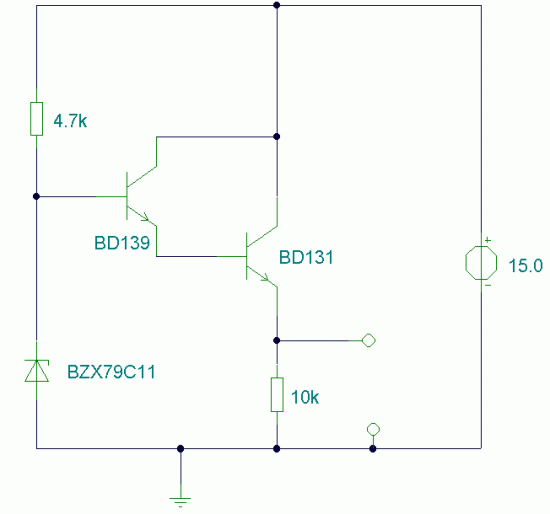## Universal DC-DC Convertor

Descriptipn:
This circuit will generate a smaller DC output voltage from a larger DC input voltage.It is quick and simple to make and by changing the value of the zener diode, the circuit can be universally adapted to provide other output voltages.The circuit and all diagrams represent a DC convertor with 12V battery input and 9Volt DC output.The output voltage is equal to the zener diode voltage less 0.7 volts, or :-

Vo = Vz - 0.7 where V z is the value of the zener diode.

With the 10V zener diode as shown in the diagram the output voltage is about 9.3 Volts DC. The supply voltage used must always be at least a few volts higher than the zener voltage. In this example I have used a 12 Volt DC battery to provide the regulated 9 Volt DC output.

Output Regulation Versus Input Voltage

The above graph shows how the output is affected by input voltage variations. This was produced with a load current of 100mA and using a 10 volt rated zener diode. Note that the circuit falls sharply out of regulation when the input voltage falls to 11.5 volt, hence the requirement for an adequate supply voltage.

Temperature Stability

Temperature stability is very good as the above graph shows. The output voltage changes by 8.5mV for every 10 degree rise in temperature. This is less than 1 mV / degree.